• 0 Vote(s) - 0 Average
• 1
• 2
• 3
• 4
• 5
Account Bound Items
#1ACCOUNT BOUND ITEMSPage link is positioned under the Profile tab and named as Account Bound Items.

1) Account bound items are permanent items that affect all your pokemon at the same time on every fight.

2) They require you to meet certain requirements to activate them.

3) When you press on the "Try to activate" button, it will show you all the requirements that you have to meet. Example:3.1) Top 10 players don't have requirements. They just need to afford the activation gold:4) Once you meet all of the requirements, you can activate the item as level 1 (paying the activation cost) and get level 1 bonus and unlock bonus (which normally is worth the item at level 20, instantly).

5) Each item can be upgraded to level 100.

5.1) After level 70, each level grants double bonus per level, making the upgrade cost worthwhile.

5.2) After level 90, Shining Amulet gives 6x bonus per level instead of 2x

6) All upgrade chances are set to 100%

7) Each item provides different bonuses:

7.1)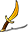Espada: Attack Bonus. Activation bonus: 2%. Level bonus (from level 1 to 70, each level): 0.1%. Level bonus (from level 71 to 100, each level): 0.2%. Total bonus (at level 100) = 15%.

7.2)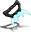Claw Blade: Special Attack Bonus. Activation bonus: 2%. Level bonus (from level 1 to 70, each level): 0.1%. Level bonus (from level 71 to 100, each level): 0.2%. Total bonus (at level 100) = 15%.

7.3)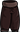Sturdy Pants: Defense Bonus. Activation bonus: 2%. Level bonus (from level 1 to 70, each level): 0.1%. Level bonus (from level 71 to 100, each level): 0.2%. Total bonus (at level 100) = 15%.

7.4)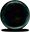Iridescent Pearl: Special Defense Bonus. Activation bonus: 2%. Level bonus (from level 1 to 70, each level): 0.1%. Level bonus (from level 71 to 100, each level): 0.2%. Total bonus (at level 100) = 15%.

7.5)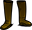Agility Boots: Speed Bonus. Activation bonus: 2%. Activation bonus: 2%. Level bonus (from level 1 to 70, each level): 0.1%. Level bonus (from level 71 to 100, each level): 0.2%. Total bonus (at level 100) = 15%.

7.6)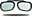Precision Spectacles: Accuracy Bonus. Activation bonus: 2%. Level bonus (from level 1 to 70, each level): 0.1%. Level bonus (from level 71 to 100, each level): 0.2%. Total bonus (at level 100) = 15%.

7.7)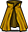Swift Cape: Evasion Chance Bonus. Activation bonus: 2%. Level bonus (from level 1 to 70, each level): 0.1%. Level bonus (from level 71 to 100, each level): 0.2%. Total bonus (at level 100) = 15%.

7.8)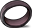Razor Ring: Critical Chance Bonus. Activation bonus: 2%. Level bonus (from level 1 to 70, each level): 0.1%. Level bonus (from level 71 to 100, each level): 0.2%. Total bonus (at level 100) = 15%.

7.9)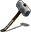Confusion Hammer: Attack and Special Attack Bonus. Activation bonus: 1%. Level bonus (from level 1 to 70, each level): 0.05%. Level bonus (from level 71 to 100, each level): 0.1%. Total bonus (at level 100) = 7.5%.

7.10)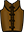Armaduras: Defense and Special Defense Bonus. Activation bonus: 1%. Level bonus (from level 1 to 70, each level): 0.05%. Level bonus (from level 71 to 100, each level): 0.1%. Total bonus (at level 100) = 7.5%.

7.11)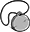Fortune Amulet: Gold boost from all NPC and Wild Pokemon battles. Activation bonus: 20%. Level bonus (from level 1 to 70, each level): 1%. Level bonus (from level 71 to 100, each level): 2%. Total bonus (at level 100) = 150%.

7.12)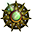Shiny Amulet: Shiny Pokémon Escape Counter Boost when searching wild Pokemon. Activation bonus: 2,000. Level bonus (from level 1 to 70, each level): 100. Level bonus (from level 71 to 100, each level): 200. Level bonus (from level 91 to 100, each level): 600. Total bonus (at level 100) = 20,000. Read more information about Escape Counters at this thread.

7.13) Egg Booster: It reduces hatching duration of your newly obtained Pokemon eggs. If you have Patron bonus they get summed. When activated, the hatching duration reduction bonus starts with 11.3% and increases 0.3% with every item level. After level 70, it gives 0.6% bonus instead of 0.3% for every level upgrade. Patron + Egg Booster can reduce egg hatching duration up to maximum 90%. When Egg Booster is level 100, it alone reduces 50% the egg hatching duration.

For example: let's say you have level 75 of this item. That means your shiny counter becomes 2,000 + 7,000 + 1,000 = 10,000. Thus, your shiny encounter chance becomes (1 + 1) / 10000 which means double chance.

Have in mind that this bonus stacks to the shiny Pokemon from the event tab. If a Pokemon has 0.05% chance of appearing, and your item is level 75, then the final chance is 0.06% instead of 0.1%.

8) All bonuses are additive instead of multiplying. So if you have bonuses from other sources (like items, account level, guild level, statuses, abilities, etc...) they are added, not multiplied.

9) User account items will be displayed in their profile.

10) The images of items are also changed based on level. Level 0 - 9 gets level 1 item image. Level 10 - 19 gets level 2 item image. Level 20 - 29 gets level 3 item image... and finally, level 100 item gets ultimate level 11 item image (epic!).

11) The amount of upgrade tries and total spent gold is displayed in the account items page and also on the user profile item image when you hover them.

11.1) Item activation cost is also included in total spent gold.

12) Account bound items are permanent and can not be destroyed, sold to other players, or traded.

12.1) You can sell your account items to NPC and get back all the gold you have spent

13) Each level upgrade cost is like below

level 0 to 1 = activation cost of each item
level 1 to 2 = 300,000
level 2 to 3 = 500,000
level 3 to 4 = 600,000
level 4 to 5 = 800,000
level 5 to 6 = 1,000,000
level 6 to 7 = 1,200,000
level 7 to 8 = 1,400,000
level 8 to 9 = 1,600,000
level 9 to 10 = 1,900,000
level 10 to 11 = 2,100,000
level 11 to 12 = 2,400,000
level 12 to 13 = 2,600,000
level 13 to 14 = 3,000,000
level 14 to 15 = 3,200,000
level 15 to 16 = 3,600,000
level 16 to 17 = 3,900,000
level 17 to 18 = 4,200,000
level 18 to 19 = 4,600,000
level 19 to 20 = 4,900,000
level 20 to 21 = 5,300,000
level 21 to 22 = 5,700,000
level 22 to 23 = 6,200,000
level 23 to 24 = 6,700,000
level 24 to 25 = 7,100,000
level 25 to 26 = 7,600,000
level 26 to 27 = 7,900,000
level 27 to 28 = 8,400,000
level 28 to 29 = 8,900,000
level 29 to 30 = 9,300,000
level 30 to 31 = 9,900,000
level 31 to 32 = 10,500,000
level 32 to 33 = 11,000,000
level 33 to 34 = 11,500,000
level 34 to 35 = 12,300,000
level 35 to 36 = 12,900,000
level 36 to 37 = 13,700,000
level 37 to 38 = 14,400,000
level 38 to 39 = 15,500,000
level 39 to 40 = 16,300,000
level 40 to 41 = 17,000,000
level 41 to 42 = 17,900,000
level 42 to 43 = 19,100,000
level 43 to 44 = 20,400,000
level 44 to 45 = 21,000,000
level 45 to 46 = 22,400,000
level 46 to 47 = 23,700,000
level 47 to 48 = 24,900,000
level 48 to 49 = 26,200,000
level 49 to 50 = 27,700,000
level 50 to 51 = 28,800,000
level 51 to 52 = 30,700,000
level 52 to 53 = 32,100,000
level 53 to 54 = 34,200,000
level 54 to 55 = 35,600,000
level 55 to 56 = 38,300,000
level 56 to 57 = 39,800,000
level 57 to 58 = 41,300,000
level 58 to 59 = 43,600,000
level 59 to 60 = 45,500,000
level 60 to 61 = 48,700,000
level 61 to 62 = 51,100,000
level 62 to 63 = 53,100,000
level 63 to 64 = 56,200,000
level 64 to 65 = 59,400,000
level 65 to 66 = 63,200,000
level 66 to 67 = 67,000,000
level 67 to 68 = 68,900,000
level 68 to 69 = 75,000,000
level 69 to 70 = 78,700,000
level 70 to 71 = 84,700,000
level 71 to 72 = 90,500,000
level 72 to 73 = 94,600,000
level 73 to 74 = 102,200,000
level 74 to 75 = 108,800,000
level 75 to 76 = 115,100,000
level 76 to 77 = 124,600,000
level 77 to 78 = 133,400,000
level 78 to 79 = 148,000,000
level 79 to 80 = 158,200,000
level 80 to 81 = 170,600,000
level 81 to 82 = 186,400,000
level 82 to 83 = 203,400,000
level 83 to 84 = 218,400,000
level 84 to 85 = 244,700,000
level 85 to 86 = 269,900,000
level 86 to 87 = 291,300,000
level 87 to 88 = 333,200,000
level 88 to 89 = 365,400,000
level 89 to 90 = 401,300,000
level 90 to 91 = 470,500,000
level 91 to 92 = 524,700,000
level 92 to 93 = 603,900,000
level 93 to 94 = 715,200,000
level 94 to 95 = 804,500,000
level 95 to 96 = 965,000,000
level 96 to 97 = 1,145,200,000
level 97 to 98 = 1,354,800,000
level 98 to 99 = 1,658,500,000
level 99 to 100 = 2,000,000,000
#2
New item :

7.13) Egg Booster: It reduces hatching duration of your newly obtained Pokemon eggs. If you have Patron bonus they get summed. When activated, the hatching duration reduction bonus starts with 11.3% and increases 0.3% with every item level. After level 70, it gives 0.6% bonus instead of 0.3% for every level upgrade. Patron + Egg Booster can reduce egg hatching duration up to maximum 90%. When Egg Booster is level 100, it alone reduces 50% the egg hatching duration.
#3
How is anyone supposed to complete:
1. Tournaments when i don't stand any chance.
3. I need of list of requirements for each item.
#4
(2021-08-11, 01:07 AM)t83uf8uf398t8u4rt Wrote: How is anyone supposed to complete:
1. Tournaments when i don't stand any chance.
3. I need of list of requirements for each item.

you dont have to win tournament battles. just battle count
#5
These game updates are what I'm looking for.
#6
thank

Forum Jump:

Users browsing this thread: 1 Guest(s)

Users browsed this thread: ajgamer5112004 , arifatdbz ,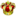CeFurkan , ch17175 , DarkKingLimon , dojeje , Fefnir , Funnygamer95 , HolyReaper1524 , jimmydean , JollyGlider97 , kane001 , Krakeder , LegnaX , lititia123 , MiguelFlores1 , Popcatmaster33 , Puka , robertsalger , SeaQueen , Soulcleaver , subject zero , t83uf8uf398t8u4rt , UnkannyKhai , Viresh Kumar , xingtian , YuetYeeHui